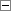Manual

# EP_TrialDays

EP_TrialDays function servers for retrieving the total number of trial period days and thenumber of trial period days left. The total days count of the trial period should be defined in TRIAL CONTROL - Limitation of days count panel. See also the extended functions EP_TrialDaysLeft and EP_TrialDaysTotal.

## Parameters

• Total - the total number of trial days.
• Left - the number of trial days left.

## Return Value

If the function succeeds, the return value is 1. If the function fails, the return value is 0.

## Remark

The function fails in the following cases:

• the limitation of the days count was not enabled;
• the application is not protected.

If the user's PC has several user accounts, the trial information will be different for each user.

## DefinitionShow/Hide C++ function definition

```extern "C" __declspec( dllimport ) __stdcall BOOL EP_TrialDays( int* Total, int* Left );
```Show/Hide Delphi function definitionShow/Hide Visual Basic function definitionShow/Hide C# (.NET) function definition

## ExamplesShow/Hide Delphi function exampleShow/Hide C++ function example

See function examples in the installation folder, Examples\Trial subfolder.